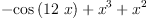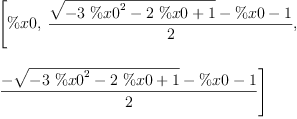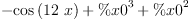login  home  contents  what's new  discussion  bug reports help  links  subscribe  changes  refresh  edit

 Submitted by : (unknown) at: 2007-11-17T22:17:39-08:00 (15 years ago) Name : Axiom Version : default friCAS-20090114 Axiom-20050901 OpenAxiom-20091012 OpenAxiom-20110220 OpenAxiom-Release-141 Category : Axiom Aldor Interface Axiom Compiler Axiom Library Axiom Interpreter Axiom Documentation Axiom User Interface building Axiom from source lisp system MathAction Doyen CD Reduce Axiom on Windows Axiom on Linux Severity : critical serious normal minor wishlist Status : open closed rejected not reproducible fix proposed fixed somewhere duplicate need more info Optional subject :   Optional comment :

In some cases FriCAS introduces special names such as %x0 standing for roots. For example:

fricas
(1) -> eq:=-cos(12*x)+x^2+x^3(1)
Type: Expression(Integer)
fricas
zerosOf(eq,x)(2)
Type: List(Expression(Integer))

That is OK and we can learn about defining equation like this:

fricas
definingPolynomial %x0(3)
Type: Expression(Integer)

However, in this case the expression (3) surfaces bug in the solver: the variable x should not appear in the defining polynomial of %x0. In fact, since the equation is not a polynomial in x zerosOf should probably reject it.

 Subject:   Be Bold !! ( 14 subscribers )